0%

In this notebook we will compare all the regression model on a dataset with 10000 values.
Models we will cover and execute a working model for:

• Multiple Linear regression
• Polynomial Regression
• RBF kernel SVR
• Decision Trees
• Random Forest

You can download the data we used in this blog by - Clicking here

Here is an entensive example of a liner regression model for refresh of your memory :)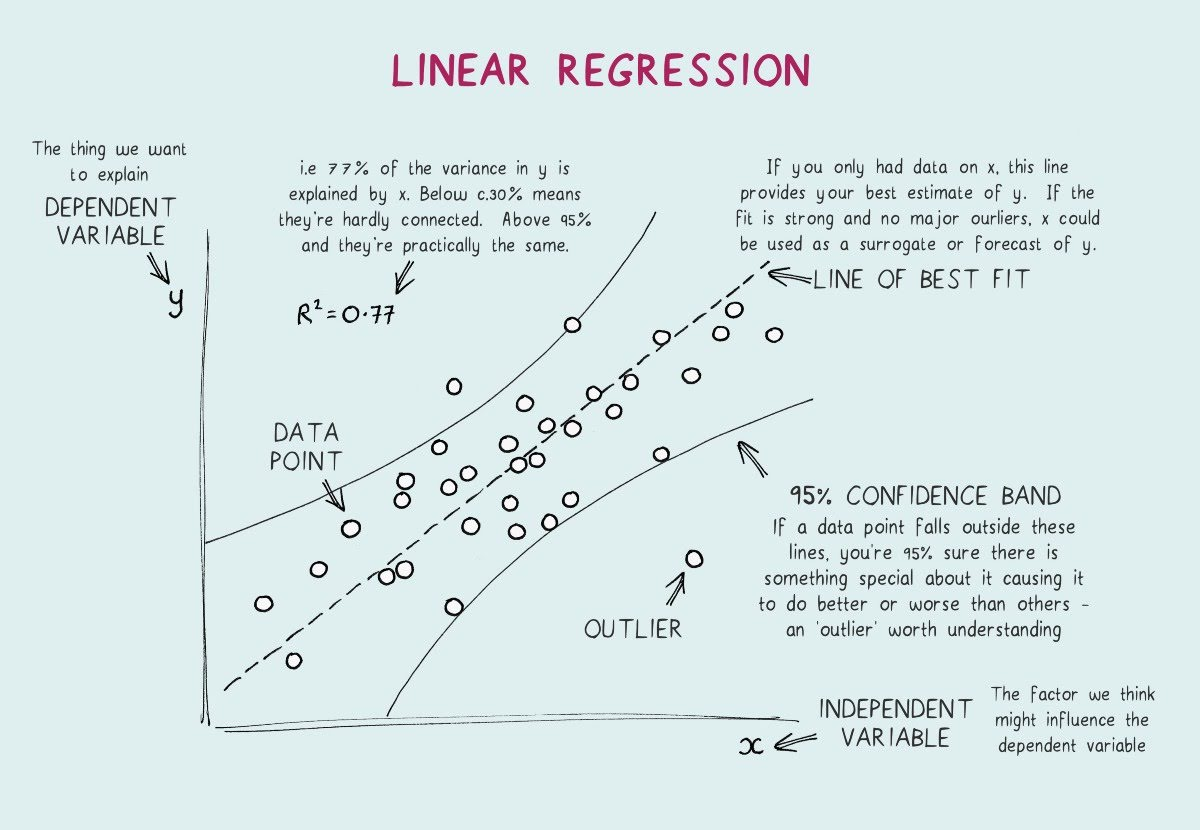## Importing libraries & reading dataset

In :
import pandas as pd
import numpy as np
from matplotlib import pyplot as plt

In :
df = pd.read_excel('00.xlsx')
df.shape #10000 rows and 5 columns

Out:
(9568, 5)
In :
df.head()

Out:
AT V AP RH PE
0 14.96 41.76 1024.07 73.17 463.26
1 25.18 62.96 1020.04 59.08 444.37
2 5.11 39.40 1012.16 92.14 488.56
3 20.86 57.32 1010.24 76.64 446.48
4 10.82 37.50 1009.23 96.62 473.90
In :
df.describe()

Out:
AT V AP RH PE
count 9568.000000 9568.000000 9568.000000 9568.000000 9568.000000
mean 19.651231 54.305804 1013.259078 73.308978 454.365009
std 7.452473 12.707893 5.938784 14.600269 17.066995
min 1.810000 25.360000 992.890000 25.560000 420.260000
25% 13.510000 41.740000 1009.100000 63.327500 439.750000
50% 20.345000 52.080000 1012.940000 74.975000 451.550000
75% 25.720000 66.540000 1017.260000 84.830000 468.430000
max 37.110000 81.560000 1033.300000 100.160000 495.760000
In :
x = df.iloc[: , 0:4].values #.values will create an array instead of a dataframe object
y = df.iloc[: , 4:5].values

In :
y.T

Out:
array([[463.26, 444.37, 488.56, ..., 429.57, 435.74, 453.28]])
In :
x.T

Out:
array([[  14.96,   25.18,    5.11, ...,   31.32,   24.48,   21.6 ],
[  41.76,   62.96,   39.4 , ...,   74.33,   69.45,   62.52],
[1024.07, 1020.04, 1012.16, ..., 1012.92, 1013.86, 1017.23],
[  73.17,   59.08,   92.14, ...,   36.48,   62.39,   67.87]])

## Split: Training & Testing

In :
from sklearn.model_selection import train_test_split
#80-20 split
x_train, x_test , y_train, y_test = train_test_split(x, y, train_size = 0.8, random_state = 0)

In :
x_train.shape

Out:
(7654, 4)
In :
x_test.shape

plt.scatter(x[:, 0],y)

Out:
<matplotlib.collections.PathCollection at 0x1a233ecf50>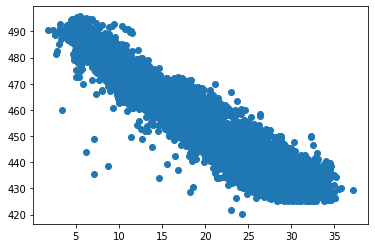## Multiple Linear Regression

In :
#doing covariance test
import statsmodels.api as sm
results = sm.OLS(y,x).fit()
print(results.summary())

                                 OLS Regression Results
=======================================================================================
Dep. Variable:                      y   R-squared (uncentered):                   1.000
Model:                            OLS   Adj. R-squared (uncentered):              1.000
Method:                 Least Squares   F-statistic:                          1.939e+07
Date:                Wed, 06 May 2020   Prob (F-statistic):                        0.00
Time:                        04:34:09   Log-Likelihood:                         -29068.
No. Observations:                9568   AIC:                                  5.814e+04
Df Residuals:                    9564   BIC:                                  5.817e+04
Df Model:                           4
Covariance Type:            nonrobust
==============================================================================
coef    std err          t      P>|t|      [0.025      0.975]
------------------------------------------------------------------------------
x1            -1.6781      0.015   -109.169      0.000      -1.708      -1.648
x2            -0.2726      0.008    -34.019      0.000      -0.288      -0.257
x3             0.5028      0.000   1209.083      0.000       0.502       0.504
x4            -0.0999      0.004    -22.678      0.000      -0.109      -0.091
==============================================================================
Omnibus:                      491.038   Durbin-Watson:                   2.021
Prob(Omnibus):                  0.000   Jarque-Bera (JB):             1475.265
Skew:                          -0.224   Prob(JB):                         0.00
Kurtosis:                       4.871   Cond. No.                         336.
==============================================================================

Warnings:
 Standard Errors assume that the covariance matrix of the errors is correctly specified.

In :
from sklearn.linear_model import LinearRegression
regressor = LinearRegression()
regressor.fit(x_train , y_train)

#print intercept and coefficient
print(regressor.coef_, '\n', regressor.intercept_)

[[-1.97 -0.24  0.06 -0.16]]
[452.84]

In :
#visualizing results
plt.figure(figsize = (10,5))
plt.scatter(x[:,0] , y )
plt.plot(x_test[:,0], regressor.predict(x_test), color = 'red')
plt.xlabel('Independent Variable')
plt.ylabel('Dependent Variable')
plt.title('Multiple Linear Regression')
plt.show()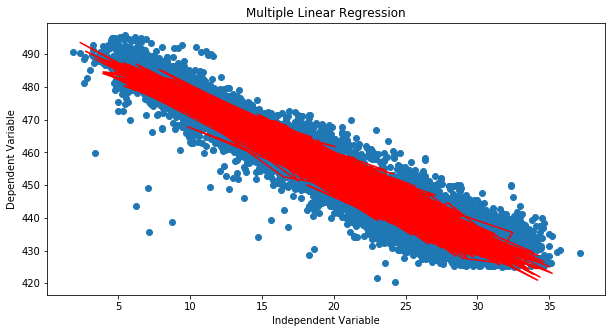### Evaluation Preformance

In :
from sklearn.metrics import r2_score
r2_score(y_test,regressor.predict(x_test))
#closer the r2 to 1, better is the score

Out:
0.9325315554761303

## Polynomial regression

In :
# importing libraries for polynomial transform
from sklearn.preprocessing import PolynomialFeatures
# for creating pipeline
from sklearn.pipeline import Pipeline
results = [('polynomial', PolynomialFeatures(degree = 5)) , ('model', LinearRegression())]
# creating pipeline and fitting it on data
pipe = Pipeline(results)
pipe.fit(x_train,y_train)

Out:
Pipeline(memory=None,
steps=[('polynomial',
PolynomialFeatures(degree=5, include_bias=True,
interaction_only=False, order='C')),
('model',
LinearRegression(copy_X=True, fit_intercept=True, n_jobs=None,
normalize=False))],
verbose=False)
In :
plt.scatter(x[:, 0],y, color = 'black')
plt.plot(x_test[:, 0], pipe.predict(x_test), color = 'purple')
plt.xlabel('Independent Variable')
plt.ylabel('Dependent Variable')
plt.title('Polynomial Regresion')
plt.show()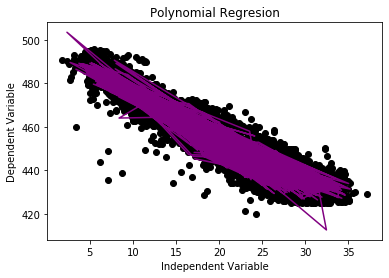### Evaluation Preformance

In :
from sklearn.metrics import r2_score
r2_score(y_test, pipe.predict(x_test))

#this score is better than multiple linear regression

Out:
0.9447852145170457

## RBF Kernel SVR

In :
from sklearn.preprocessing import StandardScaler
sc_X = StandardScaler()
sc_y = StandardScaler()
X_train = sc_X.fit_transform(x_train)
Y_train = sc_y.fit_transform(y_train)

from sklearn.svm import SVR
regressor = SVR(kernel = 'rbf')
regressor.fit(X_train, Y_train)

y_pred = sc_y.inverse_transform(regressor.predict(sc_X.transform(x_test)))
np.set_printoptions(precision=2)
print(np.concatenate((y_pred.reshape(len(y_pred),1), y_test.reshape(len(y_test),1)),1))

/Users/karan7k/opt/anaconda3/lib/python3.7/site-packages/sklearn/utils/validation.py:760: DataConversionWarning: A column-vector y was passed when a 1d array was expected. Please change the shape of y to (n_samples, ), for example using ravel().
y = column_or_1d(y, warn=True)

[[434.05 431.23]
[457.94 460.01]
[461.03 461.14]
...
[470.6  473.26]
[439.42 438.  ]
[460.92 463.28]]

In :
plt.scatter(x[:, 0],y, color = 'black')
plt.plot(x_test[:, 0], y_pred, color = 'blue')
plt.xlabel('Independent Variable')
plt.ylabel('Dependent Variable')
plt.title('Support Vector Regresion')
plt.show()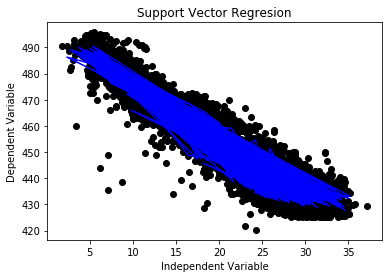In :
from sklearn.metrics import r2_score
r2_score(y_test, y_pred)

#so far the best model is SVR

Out:
0.9480784049986258

## Decision Tree Regression

In :
from sklearn.tree import DecisionTreeRegressor
treo = DecisionTreeRegressor(random_state = 0)

treo.fit(x_train , y_train)

#plotting the result after fitting
plt.scatter(x[:,0],y, color = 'purple')
plt.plot(x_test[:,0], treo.predict(x_test), color ='black')
plt.xlabel('Independent Variable')
plt.ylabel('Dependent Variable')
plt.title('Decision Tree Regression')
plt.show()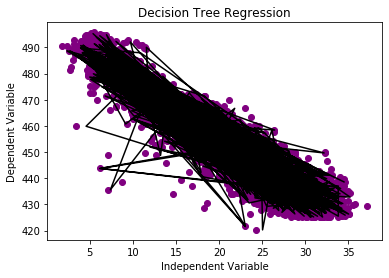### Evaluating Results

In :
from sklearn.metrics import r2_score
r2_score(y_test, treo.predict(x_test))

#so far decision tree regression is the worst model

Out:
0.9226091050550043

## Random Forest

In :
from sklearn.ensemble import RandomForestRegressor
rf = RandomForestRegressor(n_estimators = 200, random_state = 0)
rf.fit(x_train, y_train)

#plotting the result after fitting
plt.scatter(x[:,0],y, color = 'black')
plt.plot(x_test[:,0], rf.predict(x_test), color ='green')
plt.xlabel('Independent Variable')
plt.ylabel('Dependent Variable')
plt.title('Random Forest Regression')
plt.show()

/Users/karan7k/opt/anaconda3/lib/python3.7/site-packages/ipykernel_launcher.py:3: DataConversionWarning: A column-vector y was passed when a 1d array was expected. Please change the shape of y to (n_samples,), for example using ravel().
This is separate from the ipykernel package so we can avoid doing imports until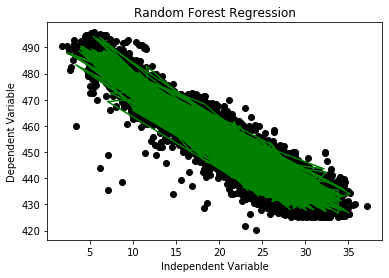### Evaluating Results

In :
from sklearn.metrics import r2_score
r2_score(y_test, rf.predict(x_test))

# Best results we have

Out:
0.9650087123017985

## Conclusion:

### Results:

NOTE: "Higher the Better"

• Multiple Linear regression - 0.9325315554761303
• Polynomial Regression - 0.9447852145170457
• RBF kernel SVR - 0.9480784049986258
• Decision Trees - 0.9226091050550043
• Random Forest - 0.9650087123017985

### Conclusion

Therefore the Random Forest Model Fits to our data well,
Considering the present scanario we will choose the random forest method for our further process

NOTE: To be considered that we have not taken to account overfitting and high variance low bais issue in this model.
Also we have not considered the hyperparameter tuning for any of the hperparameters involved.

## More Content

If you want to see the separate implementation and theory of these models on the website with a different dataset. Please click on these links below

In [ ]: